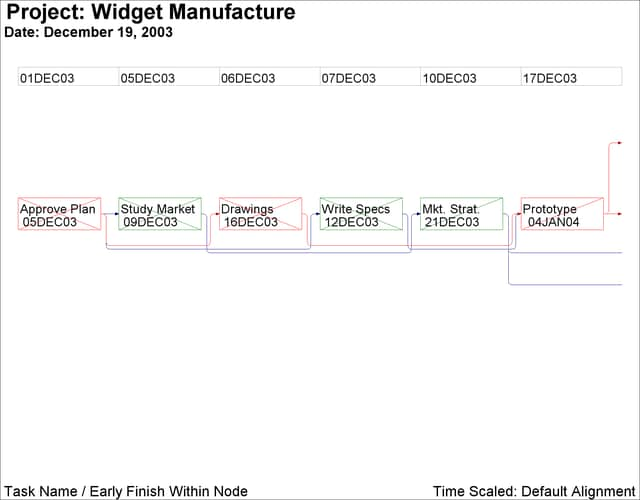### Example 9.9 Time-Scaled Network Diagram

This example illustrates the use of the TIMESCALE and ALIGN= options to draw time-scaled network diagrams. The Schedule data set `WIDGUPD`, produced by PROC CPM in the previous example, is used. First, PROC NETDRAW is invoked with the TIMESCALE option without any ALIGN= specification. By default, the procedure aligns the nodes to coincide with the early start times of the activities. The network spans two pages (HPAGES=2 VPAGES=1), as shown in Output 9.9.1. The HMARGIN= and VMARGIN= options add extra space around the margins.

```pattern1 v=e c=green;
pattern2 v=e c=red;

title  j=l h=3 ' Project: Widget Manufacture';
title2 j=l h=2 ' Date: December 19, 2003';
footnote j=l h=2 ' Task Name / Early Finish Within Node'
j=r h=2 'Time Scaled: Default Alignment ';
proc netdraw data=widgupd graphics;
succ=(succ1 succ2 succ3)
ybetween = 8
separatearcs
novcenter
nolabel
showstatus
carcs=darkblue
ccritarcs=red
vmargin=5
hmargin=5
timescale
htext=2  pcompress
hpages=2 vpages=1
nopagenumber;
run;
```

Output 9.9.1: TIMESCALE Option: Default AlignmentNext, PROC NETDRAW is invoked with several of the time-scale options:

• The ALIGN= option requests that the activities be placed according to the L_START times.

• The FRAME option produces a border around the network diagram.

• The AUTOREF option draws reference lines at every tick mark.

• The LREF= and CREF= options specify the line style and color for the reference lines.

• The SHOWBREAK option requests that breaks in the time axis be indicated by breaks before the corresponding tick marks.

```footnote j=l h=2 ' Task Name / Late Finish Within Node'
j=r h=2 'Time Scaled: Align = Late Start ';
proc netdraw data=widgupd graphics;
succ=(succ1 succ2 succ3)
ybetween = 10
separatearcs
pcompress
novcenter
nolabel
boxwidth=5
showstatus
carcs=darkcyan
ccritarcs=red
vmargin=10
align=l_start
frame
autoref
lref=33
cref=darkcyan
showbreak
htext=2;
run;
```

Output 9.9.2: Timescale Option: ALIGN= L_START